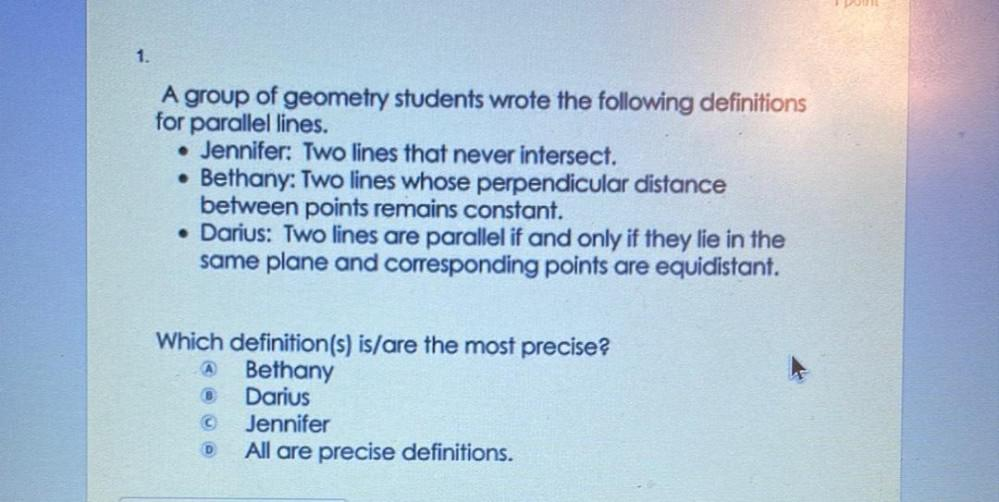Question:

# A group of geometry students wrote the following definitions for parallel lines. Jennifer: Two lines that never intersect. Bethany: Two lines whose perpendicular distance between points remains constaA group of geometry students wrote the following definitions for parallel lines. Jennifer: Two lines that never intersect. Bethany: Two lines whose perpendicular distance between points remains constant. Darius: Two lines are parallel if and only if they lie in the same plane and corresponding points are equidistant. Which definition(s) is/are the most precise? a)Bethany b)Darius c)Jennifer d)All are precise definitions.